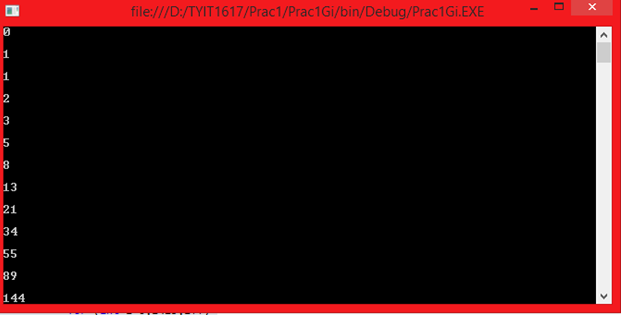Hello students over here we will learn the program of generating Fibonacci series using c#.

Explanation

1. We have declared a static function which is public in nature and the name of the function is fibo and it takes an integer variable as a parameter.
2. Inside the function we have declared 3 integer variables named num1, num2, temp.
3. We have assigned value to num 1 = 0, as we need an initial value to start with and num2 = 1 as this variable will be used to add up to num1.
4. We then run a for loop specifying the condition that it should be less than the parameter value of the function “fibo” which we have declared.
5. Inside the forloop the variable temp gets the value of num1, and num1 gets the value of num2.
6. We then set the value of num2 = the value stored in temp + the value stored in num1
7. We then return  num1.
8. Now in the main function we run another forloop and specify the condition as we like. In this example we have mentioned the end value as 15, which means the for loop with exit when the value reaches 15, mean 14 is the last value.
9. Inside the for loop we have called the static function of fibo and we have passed integer i declared in for loop of main method which in turn gets passed to the fibo method.

PDF Version here#### CONTACT US

We're not around right now. But you can send us an email and we'll get back to you, asap.

Sending

©2019 MYBSCIT.com. An initiative by some failures to make student scholars.

or